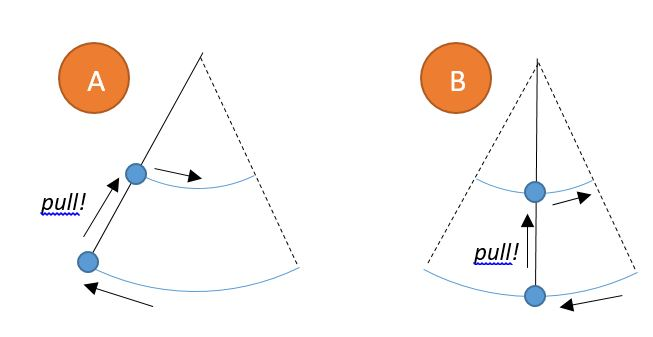# Bob Goes Faster

Scenario A: Bob attaches a mass $m$ at the end of a long string (length $L$) and holds the other end of the string, allowing the mass to swing like a pendulum, with amplitude $A$. When the mass reaches a turning point, Bob quickly pulls on the string so that the swinging part of the string shortens to $\tfrac12 L$. The pendulum now swings with a higher frequency.

Scenario B: Bob repeats the exact same situation: a pendulum length $L$ and mass $m$ is swinging with amplitude $A$. This time, Bob quickly pulls on the string when the mass passes through the equilibrium position. Again, the string is shortened to $\tfrac12 L$, and the pendulum swings with a higher frequency.

In which scenario, if any, will the pendulum have a greater amplitude after Bob shortens the string?

Assumptions: The size of the swinging mass is negligible. When Bob pulls on the string, he does this in a negligible amount of time. During the pull, the force is directed upward along the string.×

Problem Loading...

Note Loading...

Set Loading...